Mobile QR Code1. (School of Electronics Engineering, Chungbuk National University, Cheongju, 28644, Korea)
2. (Department of Computer Engineering, Chungbuk National University, Cheongju, 28644, Korea)
3. (Nano Convergence Practical Application Center, Daegu Technopark, Daegu, 42716, Korea)
4. (School of Polymer Science and Engineering, Chonnam National University, Gwangju, 61186, Korea)

Large-area OPV, flexible substrate, solution-process, low-temperature process, scalable

## I. INTRODUCTION

The installation of photovoltaic (PV) systems has been increasing rapidly owing to a rapid reduction in the cost of PV panels. Because panel costs are considered in dollars per watt, either a low cost or high performance can be beneficial (1,2). Among the many low-cost PV technologies available, organic photovoltaics (OPVs) are attracting attention owing to their low fabrication cost, printability, and simple device structure. A low fabrication cost could come not only from smaller facility costs but also a shorter fabrication time and a low thermal budget. In addition, the intrinsic nature of polymer organic materials is compatible with a flexible substrate. The portable, deformable, and stretchable nature of this flexible OPV can be used for foldable or wearable devices. A single-junction OPV achieves its best PCE of 17.35% when the area is 0.032 cm$^{2}$ (3). However, the best PCE of single-junction OPVs with an area of 1.023 and 26.129 cm$^{2}$ has decreased to 13.45% and 12.6%, respectively (3). Furthermore, the PCE of the best OPV module with an area of 802 cm$^{2}$ was 8.7% (3).

Many institutes have also investigated large-scale OPVs on top of flexible substrates. Han et al. reported an all-solution process OPV on a flexible polyethyleneterephthalate (PET) substrate with a PCE of 5.25% with an area of 80 cm$^{2}$ (4). Krebs et al. reported a roll-to-roll processed (R2R) OPV on a flexible PET substrate with a PCE of 2.1% for an active area of 120 cm$^{2}$ (5). Strohm et al. also reported a R2R processed 60 cm$^{2}$ PCE of a 5% OPV module (6).

In a previous study, a large-scale OPV was achieved from the series connection of each small-scale cell. However, in this research, we focused on the fabrication of large single cells using feasible low-cost mass-production PV fabrication methods such as low-temperature (low thermal budget), vacuum-free (time budget), and solution-based (low facility cost) processes (7,8). Various OPV sizes of 0.041–1.6 cm$^{2}$ were fabricated from a low-cost solution process for a systematic study of the loss analysis of large-scale OPVs. In addition, numerical calculations from experimental data provide a roadmap for the performance and loss of large-scale OPVs.

Fig. 1. (a) Geometrical structure, (b) FE-SEM, (c) photograph of the fabricated flexible inverted OPV.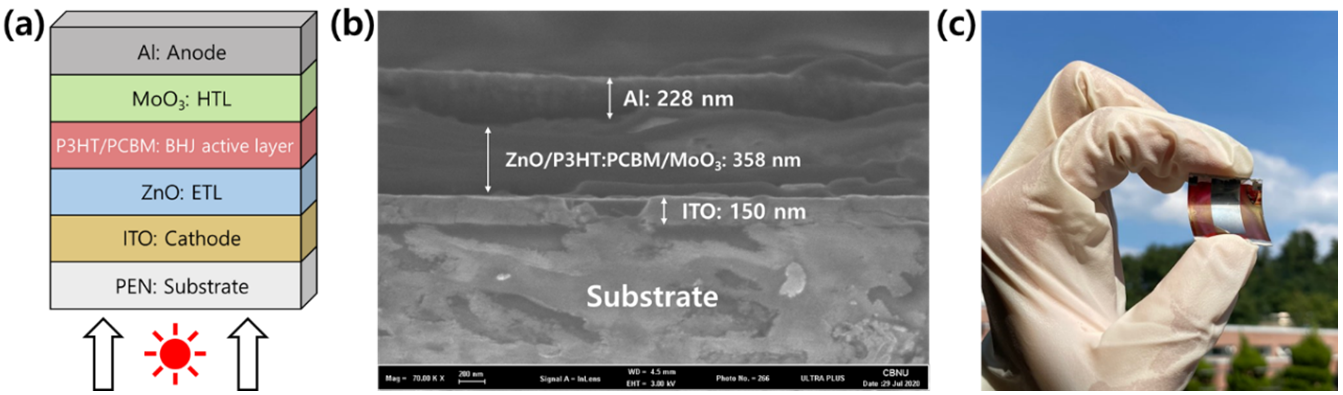## II. Experimental

The fabrication of a flexible large-area inverted solution-processed OPV starts from an indium tin oxide (ITO, 15 Ω/sq) deposited polyethylene naphthalate (PEN) substrate (thickness of 125 μm) followed by the patterning of ITO for a specific designated area. ITO patterning was conducted using a 1:1 volume ratio of deionized water to hydrochloric acid for 20 min, and a cathode electrode was applied for an inverted OPV structure (9). After ITO patterning, substrates were cleaned in a sonicator with acetone and isopropyl alcohol for 10 min. Subsequently, the ZnO layer was added to the ITO as an electron transport layer (ETL). ZnO is formed from the spin-coating of a solution containing 1.6 g of zinc acetate (Alfa Aesar, 97%) in 0.4 g of ethanolamine and 66 g of ethanol at 2000 rpm for 30 s followed by annealing at 160 $^{\circ}$C on a hot plate for 25 min (10,11). On top of the ZnO ETL layer, a 0.1 wt% polyethylenimine ethoxylated (PEIE) solution was spin-coated at 4500 rpm for 30 s and then annealed at 80 ℃ for 10 min for an interfacial buffer layer.

Subsequently, bulk heterojunction (BHJ) photoactive layers were formed from a mixed solution of 1:1 poly (3-hexylthiophene-2, 5-diyl) (P3HT, Lumtec LT-S909) and (6, 6)-Phenyl-C61 butyric acid methyl ester (PCBM, Lumtec LT-S905) in 1,2-dichlorobenzene (99% Sigma Aldrich). This solution was then spin-coated at 400 rpm for 30 s followed by annealing at 110 $^{\circ}$C for 10 min. A slightly low spin speed of 400 rpm is required for a thicker active layer to prevent pin-hole induced shunting, which may significantly degrade the solar cell performance for large areas. Finally, a hole transport layer (HTL) and an anode metal electrode were deposited from an ultra-high vacuum (10$^{-7}$ torr) thermal evaporator (Selcos CETUS OL 100). A total of 1 nm of MoO$_{3}$ and 200 nm of Al were deposited as an HTL and an anode electrode, respectively. For the reference (rigid OPV) device, all of the above processes were applied equally to a 0.7-mm thick glass substrate. Solar cell characteristic parameters were measured using a Class A AM 1.5 G spectrum solar simulator (Wacom WXS-155S-L2).

## III. Results and Discussion

### 1. Geometrical Structure of Fabricated Inverted OPVs

Fig. 1 shows the (a) geometrical structure, (b) field emission scanning electron microscopy (FE-SEM, Zeiss Ultra Plus), and (c) developed solution-processed large-area inverted OPVs on a flexible substrate (PEN). The inverted structure has an anode electrode on top and a cathode electrode on the bottom. To determine the feasibility of low-cost mass production, the reason for the adoption of the inverted structure was to fabricate OPVs under ambient air conditions, not inside a N$_{2}$ filled glove box. This is because inverted OPVs have been reported for high stability under air ambient than conventional OPVs (12). Moreover, as HTL and anode electrode are intimate in inverted OPV, so realization of all-solution process could be possible by replacing HTL and anode electrode to aqueous materials such as conductive polymer (7,8).

Fig. 2. AFM images of fabricated final OPV with (a) PEN, (b) glass substrates.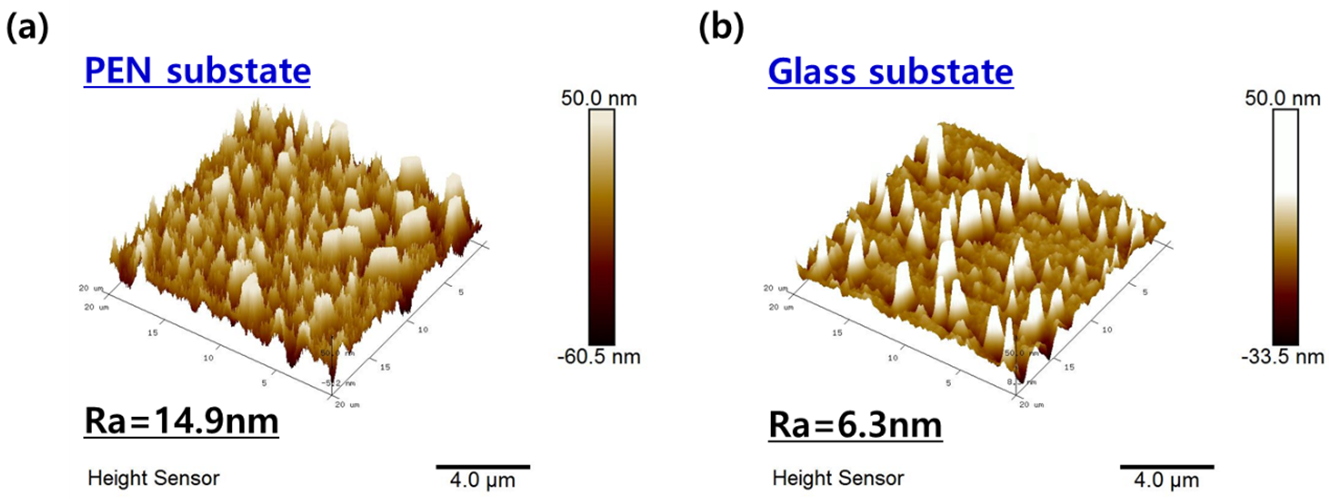The thicknesses of the cathode and anode were 150 and 228 nm, respectively, with semiconducting materials having a thickness of 358 nm. It should be noted that all processes except the anode electrode were applied from a solution with a low temperature of below 160 $^{\circ}$C, which also opens the possibility of a low thermal budget production, such as a roll-to-roll process, for this type of OPV fabrication. Note that the sample for the FE-SEM image was fabricated onto a rigid glass substrate because of the clear FE-SEM image.

Fig. 2 shows an atomic force microscopy (AFM, Bruker Dimension Icon) image of the final OPVs fabricated on glass and PEN substrates. The average roughness (Ra) of the OPV with a PEN substrate was 13.8 nm, whereas that with a glass substrate was 6.3 nm. As expected, the Ra of the OPV with the PEN substrate was higher because the rigid substrate induced an even spread of the liquid solution and easy handling.

### 2. Characteristic Parameters of Scalable Flexible Large-area Inverted Organic Photovoltaic Cells

This section evaluates the scalability of the developed solution-process flexible inverted OPVs. In addition to the PCE, the area of the solar cell is critical for its power production. One technique that cannot scale-up up to a PV module of a certain area cannot be chosen by the customer. Therefore, in this section, OPVs with different areas (0.04–1.6 cm$^{2}$) were fabricated on glass and PEN substrates to account for the impact of the area of the OPV and the rigidity of the substrate. Fig. 3 shows the normalized (a) open-circuit voltage (V$_{\mathrm{oc}}$), (b) short-circuit current density (J$_{\mathrm{sc}}$), (c) fill factor (FF), and (d) photoconversion efficiency (PCE) of the OPV with a glass or PEN substrate as a function of its area. It was found that V$_{\mathrm{oc}}$ has no significant difference for a different solar cell area. A slight degradation of the J$_{\mathrm{sc}}$ may come from the less even spread of the solution materials for larger areas owing to the limitations of the spin-coating.

A significant degradation for higher area solar cells was mainly due to FF degradation. The FF of the solar cell can be defined as Eq.(1). Where FF$_{o}$ is the ideal fill factors which does not account for parasitic resistance, v$_{oc}$ is a normalized voltage calculated from the measured V$_{\mathrm{oc}}$, and r$_{s}$ is a normalized resistance calculated from the measured R$_{\mathrm{se}}$ (series resistance), V$_{\mathrm{oc}}$, and J$_{\mathrm{sc}}$ (13). The R$_{\mathrm{se}}$ is mainly dependent on the sheet resistance (R$_{\mathrm{sh}}$) of the anode and cathode electrodes and the area of the solar cell, as shown in Eq.(2)(14). As the thickness of the organic layer (t$_{org}$) was few hundred nanometers, so other series resistance components $\left(\rho _{org}\cdot t_{org}+r_{int}\right)$ can be negligible. Therefore, R$_{\mathrm{se}}$ will be almost double when the area is double and will degrade the corresponding FF of the solar cells. It should be noted that $\rho _{org}$ and $t_{org}$ are the resistivity and thickness of the organic bulk materials, respectively, $r_{int}$ is the interface resistance. From the numerical calculations, more 1cm$^{2}$ area degrades absolute FF of ~11% and ~15% for OPV with glass and PEN substrates. As determined experimentally, an absolute FF of 10.1% occurred as the area of the solar cell increased from 0.04 to 1.0 cm$^{2}$.

Fig. 3. Normalized solar cell characteristic parameters (a) V$_{\mathrm{oc}}$, (b) J$_{\mathrm{sc}}$, (c) FF, (d) PCE as a function of the solar cell area.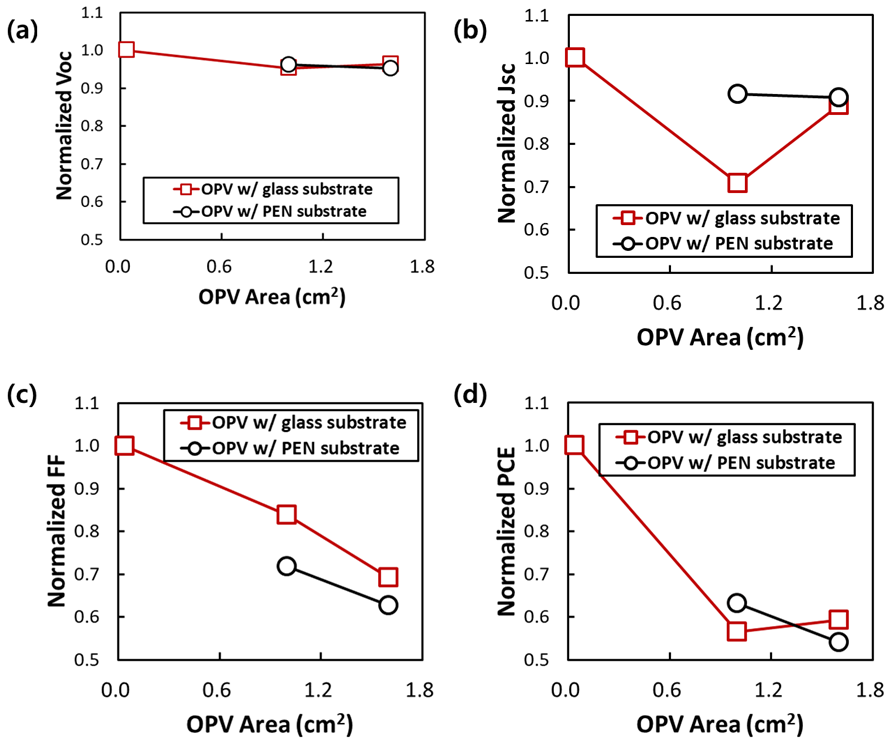Fig. 4. Calculated and experimental fill factor values as a function of the solar cell area when fabricated on both glass and PEN substrates.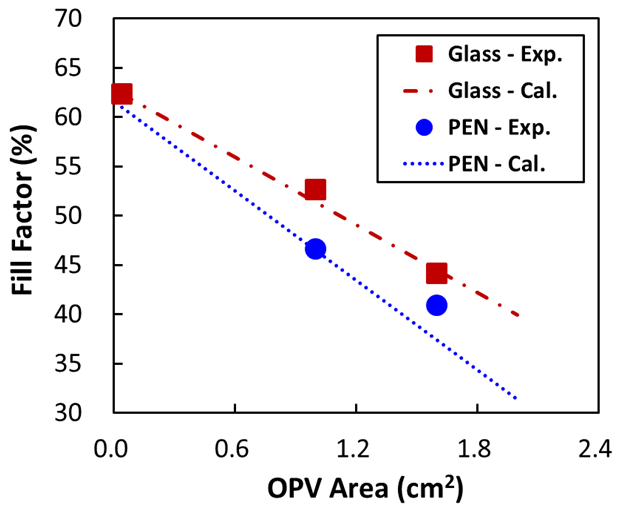##### (1)
$F F=F F_{0}\left(1-r_{s}\right), F F_{o}=\frac{v_{o c}-\ln \left(v_{o c}+0.72\right)}{v_{o c}+1}$, $v_{o c}=\frac{q V_{o c}}{n k T}, r_{s}=\frac{R_{s e}}{V_{o c} / J_{s c}}$

##### (2)
$R_{s e}=R_{s h}\left(\frac{L}{W}\right)$ Area $+\sum_{N}\left(\rho_{\text {org }} \cdot t_{\text {org }}+r_{\text {int }}\right)$

Fig. 4 shows the calculated FF as a function of the solar cell area with experimental data. Numerical calculations were conducted using Eq.(1) and (2) with input from the experimental data. It can be seen clearly that the numerical calculations matched the experimental data for both the OPV with glass and PEN substrates. Fig. 4 also provides a road map for the solar cell performance for various areas. The performance of a solar cell when scaling up from its initial performance can be anticipated. For example, if OPV area is increased from 1.0 to 3.0cm$^{2}$, FF will degrade from 52% to 30% and corresponding PCE will degrade from 2.0% to 1.2%.

Table 1. Solar cell characteristic parameters for fabricated best OPV for different areas with glass and PEN substrates

 Substrate Area ($cm^{2}$) Voc (mV) Jsc (mA/$cm^{2}$) FF (%) PCE (%) Rse (Ω-$cm^{2}$) Rsh (Ω-$cm^{2}$) Glass 1.6 601 7.5 44.0 2.0 34.6 533 PEN 1.0 604 7.7 43.2 2.0 25.9 357 1.6 599 7.6 38.9 1.8 41.5 314

Table 1 summarizes the characteristic parameters of a solar cell, and Fig. 5 shows the I-V curve of the best OPV with different areas and substrates. As the area of the OPV increased from 1.0 to 1.6 cm$^{2}$, the FF decreased from 43.2% to 38.9%, and the corresponding PCE decreased from 2.0% to 1.8%, for the PEN substrate. For reference, OPV on a glass substrate with an area of 1.6 cm$^{2}$ provided an FF of 44.0% and a PCE of 2.0%. From this result, we can conclude that a PCE degradation for higher areas is mostly from an FF degradation. Therefore, from the numerical calculations in Fig. 4, we can achieve an FF of 31.3% and PCE of 1.4% when the area increased to 2.0 cm$^{2}$. In addition, as shown in Fig. 5, the generated power of the higher area produced a higher energy proportional to its area.

## IV. CONCLUSIONS

Fig. 5. I-V curve of the fabricated best OPV for different areas with glass and PEN substrates.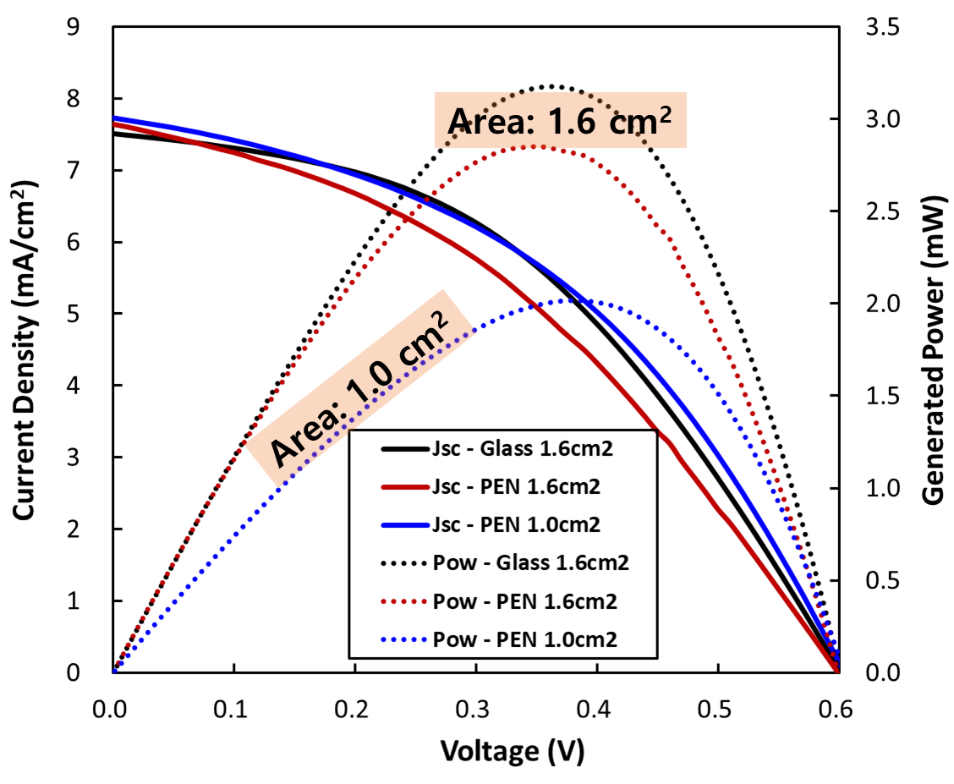Scalable inverted OPV cells were fabricated on a flexible PEN substrate. Single OPV cells with an area of over 1.6 cm$^{2}$ were fabricated using a solution process at a low temperature of below 160 $^{\circ}$C. To quantify the PCE loss for large-area solar cells and the impact of the substrate, OPVs with various areas were fabricated using a flexible PEN and rigid glass substrates. The PCE degradation of both glass and PEN substrates showed a similar trend, whereas OPV with a PEN substrate showed a slightly inferior performance. From the numerical calculations using an experimental data input, the solution-processed OPV fabrication method on a flexible substrate could have a scalability of up to an area of 2.0 cm$^{2}$ without a significant decrease in the PCE. The PCE will be 2.0%, 1.8%, and 1.4% when the area of a single OPV is 1.0, 1.6, and 2.0 cm$^{2}$, respectively.

### ACKNOWLEDGMENTS

This work was supported by the research grant of the Chungbuk National University in 2019

### REFERENCES

1
Kang M. H., Rohatgi A., Ristow A., 2014, Development of a simple analytical model to quantify the PV module cost premium associated with module efficiency and cell technology, Renew. Sustain. Energy Rev., Vol. 37, pp. 380-3852
Kang M. H., Rohatgi A., 2016, Quantitative analysis of the levelized cost of electricity of commercial scale photovoltaics systems in the US, Sol. Energy Mater. Sol. Cells, Vol. 154, pp. 71-773
Green M. A., Dunlop E. D., Hohl-Ebinger J., Yoshita M., Kopidakis N., Hao X., 2020, Solar cell efficiency tables (version 56), Prog. Photovoltaics Res. Appl., Vol. 28, No. 7, pp. 629-6384
Han Y. W., 2019, Evaporation-Free Nonfullerene Flexible Organic Solar Cell Modules Manufactured by An All-Solution Process, Adv. Energy Mater., Vol. 9, No. 42, pp. 1-155
Krebs F. C., Gevorgyan S. A., Alstrup J., 2009, A roll-to-roll process to flexible polymer solar cells: model studies, manufacture and operational stability studies, J. Mater. Chem., Vol. 19, No. 30, pp. 5442-54516
Strohm S., 2018, P3HT: Non-fullerene acceptor based large area, semi-transparent PV modules with power conversion efficiencies of 5\%, processed by industrially scalable methods, Energy Environ. Sci., Vol. 11, No. 8, pp. 2225-22347
Lee D. J., Heo D. K., Yun C., Kim Y. H., Kang M. H., 2019, Solution-Processed Semitransparent Inverted Organic Solar Cells from a Transparent Conductive Polymer Electrode, ECS J. Solid State Sci. Technol., Vol. 8, No. 2, pp. q32-Q378
Hyun D., Jae D., Kim B., Yun C., Hee M., 2020, Tailoring PEDOT:PSS polymer electrode for solution-processed inverted organic solar cells, Solid State Electron., Vol. 169, No. march, pp. 1078089
Yip H.-L., Jen A. K.-Y., 2012, Recent advances in solution-processed interfacial materials for efficient and stable polymer solar cells, Energy Environ. Sci., Vol. 5, No. 3, pp. 599410
Niesen B., Rand B. P., 2014, Thin film metal nanocluster light-emitting devices, Adv. Mater., Vol. 26, No. 9, pp. 1446-144911
Koh T.-W., 2015, Metal nanocluster light-emitting devices with suppressed parasitic emission and improved efficiency: exploring the impact of photophysical properties, Nanoscale, Vol. 7, No. 20, pp. 9140-914612
Li C. Y., 2009, An inverted polymer photovoltaic cell with increased air stability obtained by employing novel hole/electron collecting layers, J. Mater. Chem., Vol. 19, No. 11, pp. 1643-164713
Xiao X., Lee K., Forrest S. R., 2015, Scalability of multi-junction organic solar cells for large area organic solar modules, Appl. Phys. Lett., Vol. 106, No. 2114
Green M. A., 1982, Solar cells: operating principles, technology, and system applications, Prentice-Hall, Inc., Englewood Cliffs, NJ## Author

received the B.S. degree in School of Electronics Engineering, Chungbuk National University in 2021.

His research focused on the fabrication and characterization of organic solar cells.

He is currently work as a Test Engineer at the ON Semiconductor, Korea.

received the B.S. and M.S. degrees from the Department of Electrical and Electronics Engi-neering, Kyungpook National University, Korea, in 1999 and 2001, respectively, and the Ph.D. degree from the School of Electrical and Computer Engineering, Seoul National University, Korea, in 2006.

He worked on Samsung SDI Company, Korea, from 2004 to 2007.

In 2007, he was a Post-Doctoral Research Scientist with the Department of Electrical Engineering, Columbia University, New York, where he was initially engaged in research on the application of organic thin-ﬁlm transistors and new processing strategies for highly integrated organic systems.

In 2008, he joined the School of Electrical and Computer Engineering, Georgia Institute of Technology, Atlanta, GA, USA, as a Post-Doctoral Fellow working on solution-processable organic light emitting diodes.

In 2010, he was with the College of Electrical and Computer Engineering, Chungbuk National University, Cheongju, Korea, as a Professor.

His current research interests include the organic/oxide devices, ﬂexible printing electronics, large-area light sources, and energy harvesting applications.

received the B.S.(2005), M.S.(2007) and Ph.D.(2017) degree in Department of Polymer Science and Engineering from Kyungpook National University, Daegu, Korea.

His current research interests include optically active conjugated polymers, functional polymeric materials and electronic materials and devices.

was born in Mokpo, Korea, in 1979. He received the B.S. and M.S. degrees in chemistry from Korea Advanced Institute of Science and Technology (KAIST), Korea in 2000 and 2002, respectively.

In 2011, he received the Ph.D. degree in electrical engineering from the same institute for his work on optoelectronic device engineering of organic semiconductors.

From 2011 to 2013, he was a postdoctoral researcher in Institut for Angewandte Photophysik (IAPP), TU Dresden, Germany. And from 2013 to 2020, he was a research scientist in Korean government research institute, where he worked on the polymeric optoelectronic devices for cost-effective applications such as the lighting packaging or the smart label.

In August 2020, he joined the faculty of the School of Polymer Science and Engineering at Chonnan National University (Gwangju, Korea).

His major research interests include the development of a novel material and process for polymer electronics in the areas of display/lighting, energy, and electronic bio-sensor.

was born in South Korea in 1979. He received the B.S. degree in Electrical Engineering from the Korea University, Seoul, South Korea in 2005; the M.S. degree in Electrical and Computer Engineering from the University of Florida, Gainesville, FL, USA in 2007; and the Ph.D. degree in Electrical and Computer Engineering from the Georgia Institute of Technology, Atlanta, GA, USA in 2013.

He was a Senior Researcher with the Semiconductor R&D Center, Samsung Electronics, South Korea and the Center for Nano-Photonics Convergence Technology, Korea Institute of Industrial Technology, South Korea during 2013–2015 and 2015–2017, respectively.

After that, he worked with the Department of Electrical Energy Engineering, Keimyung University, South Korea as an Assistant Professor.

He is currently work as an Associate Professor with the School of Electronics Engineering, Chungbuk National University, South Korea.